Search IntMath
Close

450+ Math Lessons written by Math Professors and Teachers

5 Million+ Students Helped Each Year

1200+ Articles Written by Math Educators and Enthusiasts

Simplifying and Teaching Math for Over 23 Years

# Squaring the circle - a reader’s approach

By Murray Bourne, 12 Feb 2016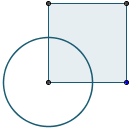The age-old problem of "squaring the circle" involves constructing a square with the same area as a given circle using only a compass and straight edge. To add to the challenge, it needs to be done in a finite number of steps.

In 1882 it was proved (as a consequence of the Lindemann–Weierstrass theorem which showed π is a transcendental number) that such a construction is impossible.

However, we can construct good approximating squares to any required level of accuracy.

I recently received a mail from Kasi Rao Jagathapu, a retired high school principal.

He wrote a paper, Squaring a Circle and Vice-versa Made Easy up to any Desired Approximation [PDF], citing the deficiencies in the "squaring the circle" constructions of Bodhayana, Jacob de Gelder and Srinivasa Ramanujan and suggesting a method for filling up the gaps.

This article outlines Kasi Rao's method (using the well-known approximation for pi of 22/7). But first, some interesting history from Kasi Rao's paper.

## History

A look into the history of Indian Mathematics reveals that in the days of Vedic civilization (1500 – 500 BCE), Indians felt that it was necessary to perform sacrificial rites to please gods and have blessings from them.

For this they needed different, specific shapes of altars or 'Vedis' of definite areas so as to make their offerings acceptable to gods. Especially when they had to construct the 'Garhapatya' altar, some opined that it should be of square shape while some others asserted that it must have circular shape. Consequently there arose the need for squaring a circle and vice versa, since in both the cases the area is to remain unaltered. Thus the impossible problem (by virtue of its involvement with the transcendental number π) squaring a circle is as old as the Vedas, the repositories of knowledge.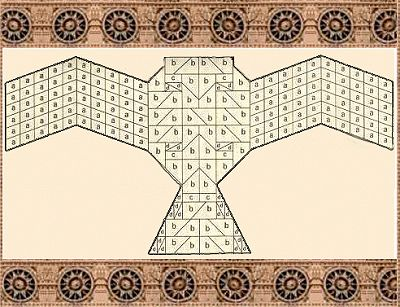A falcon-shaped Vedic altar [Image source]

Kasi Rao's paper first examines the contributions of prominent mathematicians Bodhayana (the author of the oldest Sulvasutra of Vedic age) and Srinivasa Ramanujan, the world renowned Indian Mathematician of the nineteenth century, and Jakob de Gelder (1849) towards the solution of the problem squaring a circle and vice versa.

## Kasi Rao's method for squaring the circle

The following is based on pages 5 and 6 of Kasi Rao's paper, but I'll be using 22/7 as the approximation for pi.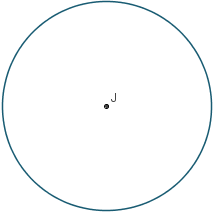Next, we construct a segment with length √7. We do that by realising 7 = 22 + 12 + 12 + 12 (the sum of four squares), and using Pythagoras' Theorem on triangles with sides of appropriate length, as follows.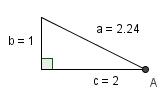The resulting length is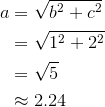Construct another right triangle, also with side length 1, as shown.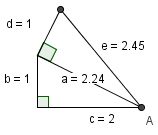The length of the segment e is found as follows: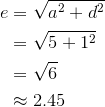One more step, and we get a segment AC: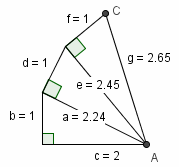The length is square root 7, as follows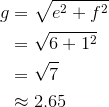We do the same on the other side, and this time we're aiming to produce a segment with length square root 22. We've used 22 = 42 + 22 + 12 + 12 and have used 3 triangles.

The result is a segment AB with length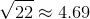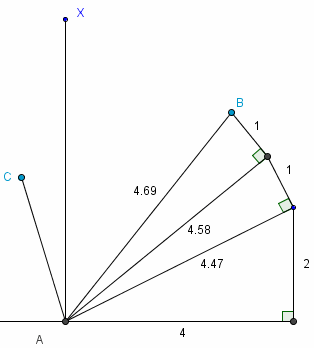Next, we construct AX, a segment which lies between AC and AB. (I've drawn it as a vertical line, but it doesn't need to be).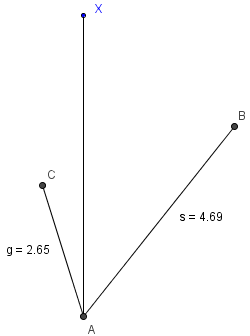Next, construct points P and Q on segments AC and AB such that AP = AQ.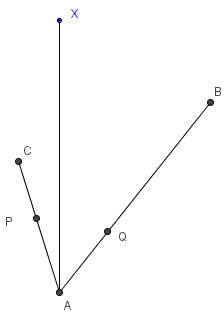Next, place point J on the segment AX and construct a circle with center J, passing through A, as follows (this is the original circle we started with, and the one for which we are trying to construct a square with the same area):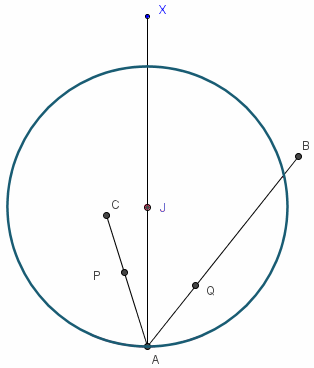Next, join CJ and contruct PL, such that CJ || PL and L is on segment AX. (The symbol || means "is parallel to".)

Similarly, join Q to L and construct a segment BK parallel to QL, as follows:We are almost done. We now construct a square with side length AK. This square will have the same area as the circle we started with (small error due to rounding).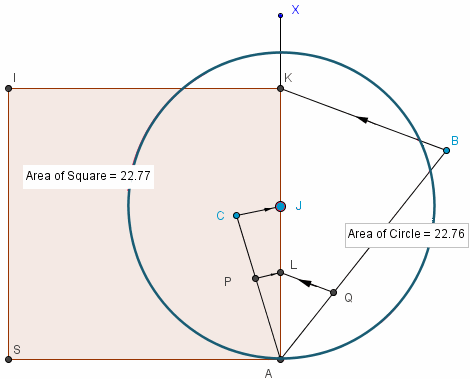I mentioned earlier AX did not need to be a vertical line. Also, the result holds for any size circle. You can see this is so in the following image, where I've moved X to the right and reduced the size of the circle: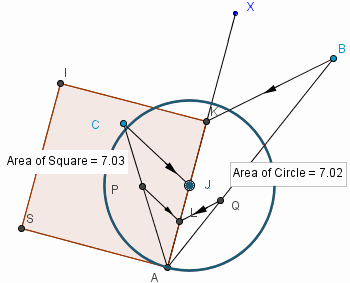## Proof the areas are equal

Since PL || CJ, triangles APL and ACJ are similar:

ΔAPL ~ ΔACJ

This means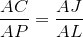We know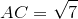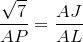Squaring both sides and rearranging gives: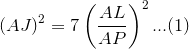Similarly, considering triangles AQL and ABK, we have:

ΔAWL ~ ΔABK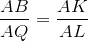Now, since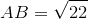, we have: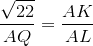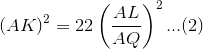Divide (2) by (1), and since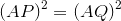then we obtain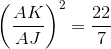This gives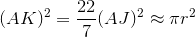So the area of the square with side AK is (approximately) the area of the circle, center J and radius AJ, both being around 22.76 m2.

## Other approximations for π

We could do the same process with other approximations for π, like: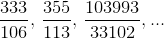We would get better approximating squares if we were to do so.

In fact, Kasi Rao's example (pages 5 and 6 of his paper) uses the second of these fractions. The difficulty level is about the same as the one I did (for 22/7), the only issue is finding the sums of squares to make 355 and 113.

In fact,

355 = 152 + 112 + 32, and

113 = 82 + 72

## Lagrange's Four-square Theorem

Lagrange's four-square theorem states that every natural number can be represented as the sum of four integer squares. [Source]

So for Kasi Rao's procedure, the most triangles we would need to construct in order to find either AB or AC is three (involving 4 segments).

## Reversibility

Kasi Rao's paper goes on to show the process can be reversed (pages 6 onwards) to find a circle with the same area as a given square ("circling the square"). The earlier historical methods were not as easy to reverse.

## Tools used

For the diagrams above I used GeoGebra. While it's not "compass and straight edge", each of the constructions could have been achieved with those tools.

## Another alternative

Jonathan Crabtree describes a neat method involving rope in How to square a circle with string.

1. Vitalie Ghelbert says:

Good morning, IntMath!

Let me please share with You some different vision regarding:

- [Quantum Continuum]: Lemma - Bijective Square The Circle - Infinite Precision [graphical method] - YouTube http://youtu.be/9MQFntVG0tM

- [Quantum Continuum]: The Divine Proportion - Absolute values - Error Zero - [All Three Hypostasis] - YouTube http://youtu.be/zySEM0LHpJo

- [Quantum Continuum]: Best Friends - Hippasus and Pythagoras - Root 2^n Order - Error Zero - YouTube http://youtu.be/niosqwrdgwM

2. Gertjan van Delft says:

Hi Murray

This easy and accurate method to circling the square is a fun one to try. I'm thinking especially for kids.
http://pidigits.ca/pidigits-squaring-the-circle.html

Regards,

Gertjan

### Comment Preview

HTML: You can use simple tags like <b>, <a href="...">, etc.

To enter math, you can can either:

1. Use simple calculator-like input in the following format (surround your math in backticks, or qq on tablet or phone):
a^2 = sqrt(b^2 + c^2)
(See more on ASCIIMath syntax); or
2. Use simple LaTeX in the following format. Surround your math with $$ and $$.
$$\int g dx = \sqrt{\frac{a}{b}}$$
(This is standard simple LaTeX.)

NOTE: You can mix both types of math entry in your comment.